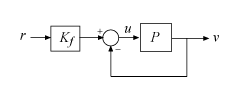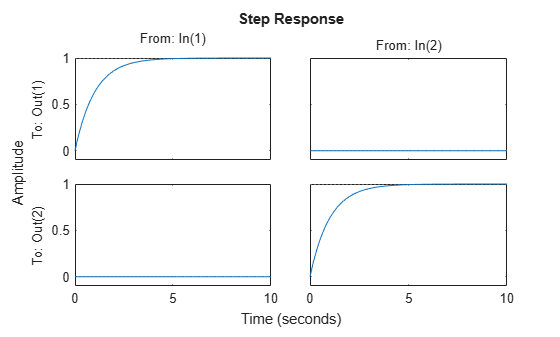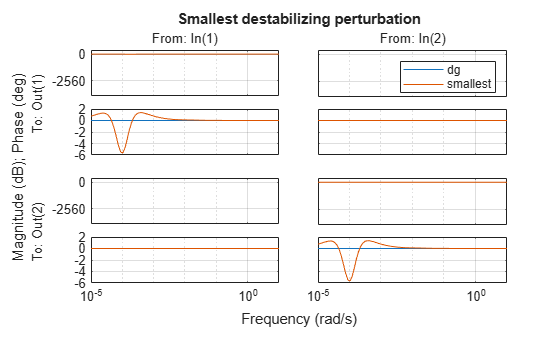Main Content

# MIMO Stability Margins for Spinning Satellite

This example shows that in MIMO feedback loops, disk margins are more reliable stability margin estimates than the classical, loop-at-a-time gain and phase margins. It first appeared in Reference .

### Spinning Satellite

This example is motivated by controlling the transversal angular rates in a spinning satellite (see Reference ). The cylindrical body spins around its axis of symmetry ($z$ axis) with constant angular rate $\Omega$. The goal is to independently control the angular rates ${\omega }_{x}$ and ${\omega }_{y}$ around the $x$ and $y$ axes using torques ${u}_{x}$ and ${u}_{y}$. The equations of motion lead to the second-order, two-input, two-output model:

`$\left(\begin{array}{c}{\underset{}{\overset{˙}{\omega }}}_{x}\\ {\underset{}{\overset{˙}{\omega }}}_{y}\end{array}\right)=\left(\begin{array}{cc}0& a\\ -a& 0\end{array}\right)\left(\begin{array}{c}{\omega }_{x}\\ {\omega }_{y}\end{array}\right)+\left(\begin{array}{c}{u}_{x}\\ {u}_{y}\end{array}\right)$`

`$\left(\begin{array}{c}{\nu }_{1}\\ {\nu }_{2}\end{array}\right)=\left(\begin{array}{cc}1& a\\ -a& 1\end{array}\right)\left(\begin{array}{c}{\omega }_{x}\\ {\omega }_{y}\end{array}\right),\phantom{\rule{0.2777777777777778em}{0ex}}\phantom{\rule{0.2777777777777778em}{0ex}}\phantom{\rule{0.2777777777777778em}{0ex}}a=10.$`

Consider a static control law that combines feedforward and unit-feedback actions:

$u={K}_{f}r-\nu$.

Setting the feedforward gain as follows achieves perfect decoupling and makes each loop respond as a first-order system with unit time constant.

`${K}_{f}=\frac{1}{1+{a}^{2}}\left(\begin{array}{cc}1& -a\\ a& 1\end{array}\right)$`

The resulting system is the two-loop control system of the following diagram.Create a state-space model of the satellite and the controller to see the performance of the system with this controller.

```% Plant a = 10; A = [0 a;-a 0]; B = eye(2); C = [1 a;-a 1]; D = 0; P = ss(A,B,C,D); % Prefilter Kf = [1 -a;a 1]/(1+a^2); % Closed-loop model T = feedback(P,eye(2))*Kf; step(T,10)```### Loop-at-a-Time Gain and Phase Margins

The classical gain and phase margins are a customary way to gauge the robustness of SISO feedback loops to plant uncertainty. In MIMO control systems, the gain and phase margins are often assessed one loop at a time, meaning that the margins are computed independently for each feedback channel with the other loops closed. Robustness is clearly in doubt when one loop is found to have weak margins. Unfortunately, the converse is not true. Each individual loop can have strong gain and phase margins while overall robustness is weak. This is because the loop-at-a-time approach fails to account for loop interactions and conditioning of the MIMO loop transfer. The spinning satellite example is a perfect illustration of this point.

To compute the loop-at-a-time gain and phase margins, introduce an analysis point at the plant input to facilitate access to the open-loop responses.

```uAP = AnalysisPoint('u',2); T = feedback(P*uAP,eye(2))*Kf;```

Use `getLoopTransfer` to access the SISO loop transfers at ${u}_{x}$ with the $y$ loop closed, and at ${u}_{y}$ with the $x$ loop closed.

```Lx = getLoopTransfer(T,'u(1)',-1); Ly = getLoopTransfer(T,'u(2)',-1);```

Finally, use `margin` to compute the loop-at-a-time classical gain and phase margins.

`[gmx,pmx] = margin(Lx)`
```gmx = Inf ```
```pmx = 90 ```
`[gmy,pmy] = margin(Ly)`
```gmy = Inf ```
```pmy = 90 ```

`margin` reports a gain margin of `Inf` and phase margin of 90°, as stable against variations in gain and phase as a SISO loop can be. This result is not surprising given that the loop transfers `Lx` and `Ly` are pure integrators.

`tf(Lx), tf(Ly)`
```ans = From input "u(1)" to output "u(1)": 1 - s Continuous-time transfer function. ans = From input "u(2)" to output "u(2)": 1 - s Continuous-time transfer function. ```

Despite this loop-at-a-time result, the MIMO feedback loop T can be destabilized by a 10% gain change affecting both loops. To demonstrate this destabilization, multiply the plant by a static gain that decreases the gain by 10% in the ${u}_{x}$ channel and increases it by 10% in the ${u}_{y}$ channel. Then compute the poles of the unit feedback loop to see that one of them has moved into the right half-plane, driving the loop unstable.

```dg = diag([1-0.1,1+0.1]); pole(feedback(P*dg,eye(2)))```
```ans = 2×1 -2.0050 0.0050 ```

Because model uncertainty typically affects all feedback loops, the loop-at-a-time margins tend to overestimate actual robustness and can miss important issues with MIMO designs.

### Disk Margins

The notion of disk margin gives a more comprehensive assessment of robustness in MIMO feedback loops by explicitly modeling and accounting for loop interactions. See Stability Analysis Using Disk Margins for additional background information. To compute the SISO and MIMO disk margins for the spinning satellite example, extract the MIMO loop transfer `L` and use the `diskmargin` command.

```L = getLoopTransfer(T,'u',-1); [DM,MM] = diskmargin(L);```

The first output `DM` contains the loop-at-a-time disk-based margins. These are lower estimates of the classical gain and phase margins. For this model, the disk-based margins also report a gain margin of `Inf` and phase margin of 90° for each individual loop. So far nothing new.

`DM(1)`
```ans = struct with fields: GainMargin: [0 Inf] PhaseMargin: [-90 90] DiskMargin: 2 LowerBound: 2 UpperBound: 2 Frequency: 0 WorstPerturbation: [2x2 ss] ```
`DM(2)`
```ans = struct with fields: GainMargin: [0 Inf] PhaseMargin: [-90 90] DiskMargin: 2 LowerBound: 2 UpperBound: 2 Frequency: 0 WorstPerturbation: [2x2 ss] ```

The second output `MM` contains the MIMO disk-based margins.

`MM`
```MM = struct with fields: GainMargin: [0.9051 1.1049] PhaseMargin: [-5.7060 5.7060] DiskMargin: 0.0997 LowerBound: 0.0997 UpperBound: 0.0999 Frequency: 1.0000e-04 WorstPerturbation: [2x2 ss] ```

This multiloop disk-based margin is the most reliable measure of MIMO robustness since it accounts for independent gain and phase variations in all loop channels at the same time. Here `MM` reports a gain margin of [0.905,1.105], meaning that the open-loop gain can increase or decrease by a factor of 1.105 while preserving closed-loop stability. The phase margin is 5.7°. This result is in line with the destabilizing perturbation `dg` above which corresponds to a relative gain change in the range [0.90,1.10].

The `diskmarginplot` command lets you visualize the disk-based margins as a function of frequency. For this system, that the disk-based margins are weakest near DC (zero frequency).

```clf diskmarginplot(L) grid```The struct `MM` also contains the smallest destabilizing perturbation `MM.WorstPerturbation`. This dynamic perturbation is a realization of the smallest destabilizing change in gain and phase. Note that this perturbation modifies both gain and phase in both feedback channels.

```WGP = MM.WorstPerturbation; pole(feedback(P*WGP,eye(2)))```
```ans = 8×1 complex -1.0000 + 0.0001i -1.0000 - 0.0001i -0.0002 + 0.0002i -0.0002 - 0.0002i -0.0000 + 0.0001i -0.0000 - 0.0001i -0.0000 + 0.0000i -0.0000 - 0.0000i ```

Compare the dynamic perturbation `WGP` with the static gain `dg` used above to destabilize the system.

```bode(ss(dg),WGP), grid title('Smallest destabilizing perturbation') legend('dg','smallest')```### Conclusion

For multi-loop control systems, disk margins are a more reliable robust stability test than loop-at-a-time gain and phase margins. The `diskmargin` function computes both loop-at-a-time and MIMO disk margins and the corresponding smallest destabilizing perturbations. You can use these perturbations for further analysis and risk assessment, for example, by injecting them in a detailed nonlinear simulation of the system.

To learn how to design a controller with adequate stability margins for this plant, see the example Robust Controller for Spinning Satellite.

### References

 Doyle, J. “Robustness of Multiloop Linear Feedback Systems.” In 1978 IEEE Conference on Decision and Control Including the 17th Symposium on Adaptive Processes, 12–18. San Diego, CA, USA: IEEE, 1978. https://doi.org/10.1109/CDC.1978.267885.

 Zhou, K., J.C.Doyle, and K. Glover. Robust and Optimal Control, Englewood Cliffs, NJ: Prentice-Hall, 1996, Section 9.6.

## SupportGet trial now